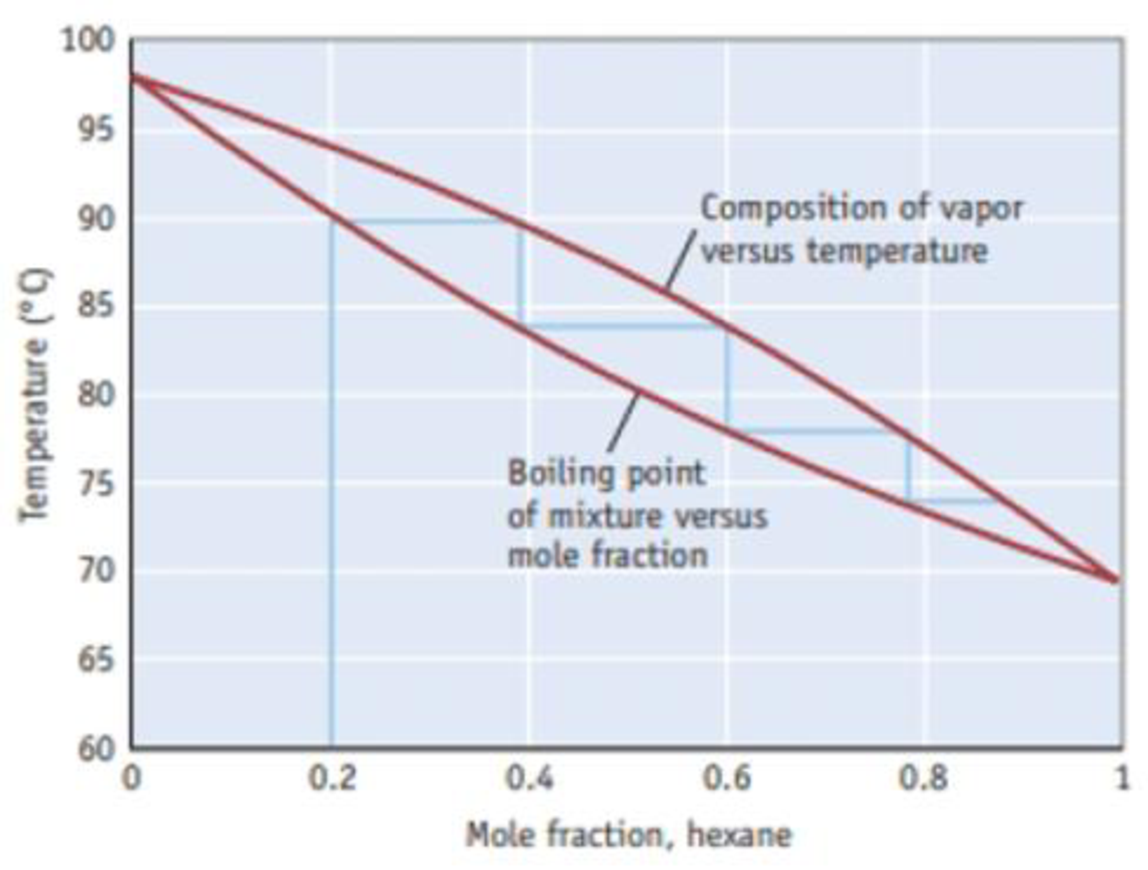# How many theoretical plates are required to produce a solution with a mole fraction of hexane greater than 0.90? Figure B A temperature-composition diagram for hexane (C 6 H 14 )-heptane (C 7 H 16 ) mixtures.### Chemistry & Chemical Reactivity

9th Edition
John C. Kotz + 3 others
Publisher: Cengage Learning
ISBN: 9781133949640

#### Solutions

Chapter
Section### Chemistry & Chemical Reactivity

9th Edition
John C. Kotz + 3 others
Publisher: Cengage Learning
ISBN: 9781133949640
Chapter 13.5, Problem 2Q
Textbook Problem
51 views

## How many theoretical plates are required to produce a solution with a mole fraction of hexane greater than 0.90?Figure B A temperature-composition diagram for hexane (C6H14)-heptane (C7H16) mixtures.

Interpretation Introduction

Interpretation: By using the given temperature-composition diagram it should be determined that how many theoretical plates are required to produce a solution with a mole fraction of hexane greater than0.90.

Concept introduction:

• Mole fraction is one of the concentrations expressing term which is equal to the number of moles of a component divided by the total number of a solution. The mole fraction of all components of a solution, when added together will equal to 1
• Fractional distillation it is a separation process in which the volatile components of a mixture are split from one another by heating the mixture in a column and collecting and condensing the vapors drawn from different levels of the column.
• The efficiency of fractional distillation column is expressed in terms of theoretical plate. Each evaporation-condensation cycles requires one theoretical plate. The greater the number of theoretical plates the better the separation for most mixtures.

### Explanation of Solution

Temperature – composition diagram for hexane (C6H14) – heptane (C7H16) mixtures.

Here the lower curved line indicates the boiling point of the mixture as a function of mole fraction of the more volatile component, hexane. The upper curve indicates the mole fraction of hexane in the vapor phase above the boiling solution. The blue line represents the change in composition of the mixture in the course of the fractional distillation of a mixture with an initial hexane mole fraction of0

### Still sussing out bartleby?

Check out a sample textbook solution.

See a sample solution

#### The Solution to Your Study Problems

Bartleby provides explanations to thousands of textbook problems written by our experts, many with advanced degrees!

Get Started

Find more solutions based on key concepts
What are some cell responses to signals?

Biology (MindTap Course List)

Chylomicrons, a class of lipoprotein, are produced in the live. T F

Nutrition: Concepts and Controversies - Standalone book (MindTap Course List)

What is the structure and function of the extracellular matrix?

Biology: The Dynamic Science (MindTap Course List)

When alcohol is rubbed on your body, it lowers skin temperature. Explain this effect.

Physics for Scientists and Engineers, Technology Update (No access codes included)

How can wind-driven horizontal movement of water induce vertical movement in surface water?

Oceanography: An Invitation To Marine Science, Loose-leaf Versin